# Convert the column type from string to datetime format in Pandas dataframe

• Difficulty Level : Basic
• Last Updated : 05 Oct, 2020

While working with data in Pandas, it is not an unusual thing to encounter time series data, and we know Pandas is a very useful tool for working with time-series data in python.
Let’s see how we can convert a dataframe column of strings (in dd/mm/yyyy format) to datetime format. We cannot perform any time series based operation on the dates if they are not in the right format. In order to be able to work with it, we are required to convert the dates into the datetime format.

Code #1 : Convert Pandas dataframe column type from string to datetime format using pd.to_datetime() function.

## Python3

 `# importing pandas as pd` `import` `pandas as pd`   `# Creating the dataframe` `df ``=` `pd.DataFrame({``'Date'``:[``'11/8/2011'``, ``'04/23/2008'``, ``'10/2/2019'``],` `                ``'Event'``:[``'Music'``, ``'Poetry'``, ``'Theatre'``],` `                ``'Cost'``:[``10000``, ``5000``, ``15000``]})`   `# Print the dataframe` `print``(df)`   `# Now we will check the data type ` `# of the 'Date' column` `df.info()`

Output: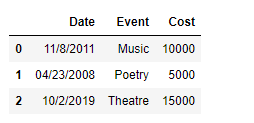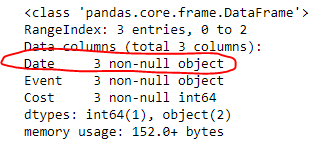As we can see in the output, the data type of the ‘Date’ column is object i.e. string. Now we will convert it to datetime format using pd.to_datetime() function.

## Python3

 `# convert the 'Date' column to datetime format` `df[``'Date'``]``=` `pd.to_datetime(df[``'Date'``])`   `# Check the format of 'Date' column` `df.info()`

Output: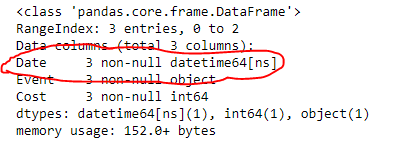As we can see in the output, the format of the ‘Date’ column has been changed to the datetime format.

Code #2: Convert Pandas dataframe column type from string to datetime format using DataFrame.astype() function.

## Python3

 `# importing pandas as pd` `import` `pandas as pd`   `# Creating the dataframe` `df ``=` `pd.DataFrame({``'Date'``:[``'11/8/2011'``, ``'04/23/2008'``, ``'10/2/2019'``],` `                ``'Event'``:[``'Music'``, ``'Poetry'``, ``'Theatre'``],` `                ``'Cost'``:[``10000``, ``5000``, ``15000``]})`   `# Print the dataframe` `print``(df)`   `# Now we will check the data type` `# of the 'Date' column` `df.info()`

Output :As we can see in the output, the data type of the ‘Date’ column is object i.e. string. Now we will convert it to datetime format using DataFrame.astype() function.

## Python3

 `# convert the 'Date' column to datetime format` `df[``'Date'``] ``=` `df[``'Date'``].astype(``'datetime64[ns]'``)`   `# Check the format of 'Date' column` `df.info()`

Output :As we can see in the output, the format of the ‘Date’ column has been changed to the datetime format.

Code #3: If the data frame column is in ‘yymmdd’ format and we have to convert it to ‘yyyymmdd’ format

## Python3

 `# importing pandas library` `import` `pandas as pd`   `# Initializing the nested list with Data set` `player_list ``=` `[[``'200712'``,``50000``],[``'200714'``,``51000``],[``'200716'``,``51500``],` `            ``[``'200719'``,``53000``],[``'200721'``,``54000``],` `            ``[``'200724'``,``55000``],[``'200729'``,``57000``]]`   `# creating a pandas dataframe` `df ``=` `pd.DataFrame(player_list,columns``=``[``'Dates'``,``'Patients'``])`   `# printing dataframe ` `print``(df)` `print``()`   `# checking the type ` `print``(df.dtypes)`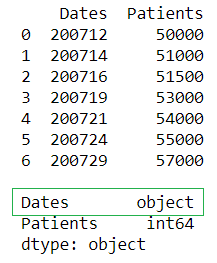## Python3

 `# converting the string to datetime format ` `df[``'Dates'``] ``=` `pd.to_datetime(df[``'Dates'``], ``format``=``'%y%m%d'``) `   `# printing dataframe ` `print``(df)` `print``()`   `print``(df.dtypes)`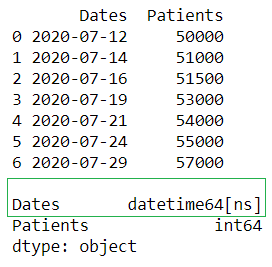In the above example, we change the data type of column ‘Dates’ from ‘object‘ to ‘datetime64[ns]‘ and format from ‘yymmdd’ to ‘yyyymmdd’.

Code #4: Converting multiple columns from string to ‘yyyymmdd‘ format using pandas.to_datetime()

## Python3

 `# importing pandas library` `import` `pandas as pd`   `# Initializing the nested list with Data set` `player_list ``=` `[[``'20200712'``,``50000``,``'20200812'``],` `               ``[``'20200714'``,``51000``,``'20200814'``],` `               ``[``'20200716'``,``51500``,``'20200816'``],` `               ``[``'20200719'``,``53000``,``'20200819'``],` `               ``[``'20200721'``,``54000``,``'20200821'``],` `               ``[``'20200724'``,``55000``,``'20200824'``],` `               ``[``'20200729'``,``57000``,``'20200824'``]]`   `# creating a pandas dataframe` `df ``=` `pd.DataFrame(` `  ``player_list,columns ``=` `[``'Treatment_start'``,` `                         ``'No.of Patients'``,` `                         ``'Treatment_end'``])`   `# printing dataframe ` `print``(df)` `print``()`   `# checking the type ` `print``(df.dtypes)`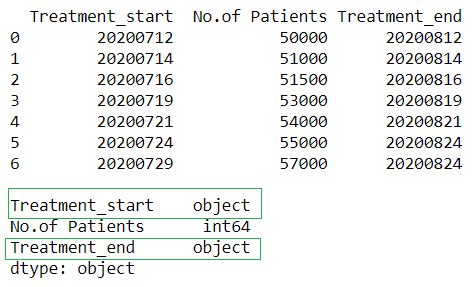## Python3

 `# converting the string to datetime` `# format in multiple columns` `df[``'Treatment_start'``] ``=` `pd.to_datetime(` `                          ``df[``'Treatment_start'``], ` `                          ``format``=``'%Y%m%d'` `) ` `df[``'Treatment_end'``] ``=` `pd.to_datetime(` `                          ``df[``'Treatment_end'``], ` `                          ``format``=``'%Y%m%d'` `) `     `# printing dataframe ` `print``(df)` `print``()`   `print``(df.dtypes)`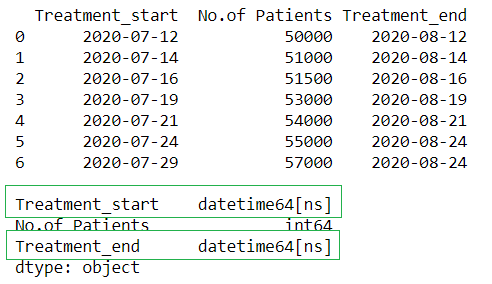In the above example, we change the data type of columns ‘Treatment_start‘ and ‘Treatment_end‘ from ‘object‘ to ‘datetime64[ns]‘ type.

My Personal Notes arrow_drop_up
Recommended Articles
Page :